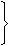# Aptitude - Chain Rule

Exercise : Chain Rule - General Questions
1.
3 pumps, working 8 hours a day, can empty a tank in 2 days. How many hours a day must 4 pumps work to empty the tank in 1 day?
9
10
11
12
Explanation:

Let the required number of working hours per day be x.

More pumps, Less working hours per day (Indirect Proportion)

Less days, More working hours per day (Indirect Proportion)

 Pumps 4 : 3:: 8 : x Days 1 : 24 x 1 x x = 3 x 2 x 8x = (3 x 2 x 8) (4)x = 12.

2.
If the cost of x metres of wire is d rupees, then what is the cost of y metres of wire at the same rate?
 Rs.xyd
Rs. (xd)
Rs. (yd)
 Rs.ydx
Explanation:

Cost of x metres = Rs. d.

 Cost of 1 metre = Rs.dx

 Cost of y metres = Rs.d . y= Rs.yd. x x

3.
Running at the same constant rate, 6 identical machines can produce a total of 270 bottles per minute. At this rate, how many bottles could 10 such machines produce in 4 minutes?
648
1800
2700
10800
Explanation:

Let the required number of bottles be x.

More machines, More bottles (Direct Proportion)

More minutes, More bottles (Direct Proportion)

 Machines 6 : 10:: 270 : x Time (in minutes) 1 : 46 x 1 x x = 10 x 4 x 270x = (10 x 4 x 270) (6)x = 1800.

4.
A fort had provision of food for 150 men for 45 days. After 10 days, 25 men left the fort. The number of days for which the remaining food will last, is:
 29 1 5
 37 1 4
42
54
Explanation:

After 10 days : 150 men had food for 35 days.

Suppose 125 men had food for x days.

Now, Less men, More days (Indirect Proportion)125 : 150 :: 35 : x125 x x = 150 x 35x = 150 x 35 125x = 42.

5.
39 persons can repair a road in 12 days, working 5 hours a day. In how many days will 30 persons, working 6 hours a day, complete the work?
10
13
14
15
Explanation:

Let the required number of days be x.

Less persons, More days (Indirect Proportion)

More working hours per day, Less days (Indirect Proportion)

 Persons 30 : 39:: 12 : x Working hours/day 6 : 530 x 6 x x = 39 x 5 x 12x = (39 x 5 x 12) (30 x 6)x = 13.